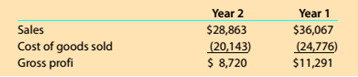# Gross profit percent and markup percent Deere &amp; Company (DE) produces and sells tractors, loaders, combines, lawnmowers, and a variety of other equipment. The following data (in millions) were adapted from recent financial statements of Deere. Compare the results in parts (1) and (2) for ‘tears 1 and 2. Comment on your comparison.### Survey of Accounting (Accounting I)

8th Edition
Carl Warren
Publisher: Cengage Learning
ISBN: 9781305961883

#### Solutions

Chapter
Section### Survey of Accounting (Accounting I)

8th Edition
Carl Warren
Publisher: Cengage Learning
ISBN: 9781305961883
Chapter 4, Problem 4.7.3MBA
Textbook Problem
1 views

## Gross profit percent and markup percent Deere & Company (DE) produces and sells tractors, loaders, combines, lawnmowers, and a variety of other equipment. The following data (in millions) were adapted from recent financial statements of Deere.Compare the results in parts (1) and (2) for ‘tears 1 and 2. Comment on your comparison.

To determine

Concept Introduction:

Gross Profit ratio:

Gross profit ratio is calculated by dividing the gross profit by sales. The formula to calculate the gross profit ratio is as follows:

Gross Profit = Gross ProfitSales

To Indicate:

The comparison for year 2 and year 1

### Explanation of Solution

The Gross Profit % for Years 1 and 2 are calculated as follows:

 Year 2 Year 1 Gross Profit (A) $8,720$ 11,291 Sales (B) $28,863$ 36,067 Gross Profit (% = (A/B) 30.2% 31...

### Still sussing out bartleby?

Check out a sample textbook solution.

See a sample solution

#### The Solution to Your Study Problems

Bartleby provides explanations to thousands of textbook problems written by our experts, many with advanced degrees!

Get Started